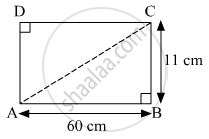Share

# Find the Length a Diagonal of a Rectangle Having Sides 11 Cm and 60 Cm. - Geometry

ConceptApollonius Theorem

#### Question

Find the length a diagonal of a rectangle having sides 11 cm and 60 cm.

#### SolutionAccording to Pythagoras theorem,
In ∆ABC

${AB}^2 + {BC}^2 = {AC}^2$
$\Rightarrow \left( 60 \right)^2 + \left( 11 \right)^2 = {AC}^2$
$\Rightarrow 3600 + 121 = {AC}^2$
$\Rightarrow {AC}^2 = 3721$
$\Rightarrow AC = 61 cm$

Hence, the length of a diagonal of the rectangle is 61 cm.

Is there an error in this question or solution?

#### APPEARS IN

Solution Find the Length a Diagonal of a Rectangle Having Sides 11 Cm and 60 Cm. Concept: Apollonius Theorem.
S